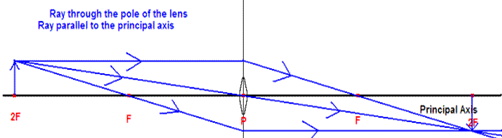Draw a figure explaining various terms related to a lens.

Question 2.

Draw a figure explaining various terms related to a lens.

i. OpticalCentre:

The center point of a lens that lies on the principal axis of the lens is called its optical center. The optical center is represented by letter C.

ii. Principal Axis

The principal axis is a straight line passing through the optical center and the center of curvature of two surfaces of a lens. It is represented by letter P

iii. Principal Focus

The principal focus is a point on its principal axis to which the light rays parallel to the principal axis converge (in case of convex lens) or appear to diverge (in case of the concave lens) after passing through it. It is represented by letter F

iv. Focal Length

The focal length of a lens is the distance between its optical center and principal focus. It is represented by letter f

F = Principal focus

2F= Pole of the lens

C = Optical centre

P = Principal axis